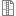# 17ABBMS - Modelling and Simulation

Code Completion Credits Range Language
17ABBMS Z,ZK 4 2P+2C English
Introduction to Signals and Systems (17ABBUSS)
Lecturer:
Jan Kauler
Tutor:
Jan Kauler
Supervisor:
Department of Biomedical Informatics
Synopsis:

Basic concepts. Aims and consequences of modeling and simulation. The methodology of modeling and simulation. Inverse problem. Proposal for a new, respectively. additional experiment. Compartmental models. Physiological models. Pharmacokinetics. Continuous and discrete models of population dynamics. Epidemiological models. Veneral disease models.

Requirements:

Knowledge of diffrential and integral calculus and theory of signals and systems.

Requirements:

1. Assignment (min. 50 % points) includes - test (max. 20 pts. on 10th Practice)

- course project (max. 20 pts. - implementation + presentation (last practice))

--&gt; 7 pts. for design, 7 implementation, 3 presentation, 3 quality of the solution

2. Exam - written exam (max. 60 pts.)

- pass the exam - min 50 % of pts. (= 30 pts.)

Final grade (ECTS scale) - exam + practices

Syllabus of lectures:

1. Basic concepts of simulation. Aims and consequences of modeling and simulation. The methodology of model development. Parameter identification. Experiments. Objective reality, dynamical systems, mathematical and simulation. Models and their description. Informal and formal description. Forms of mathematical description of continuous and discrete systems.

2. Continuous and discrete models of single populations. Malthus continuous model. Continuous logistic model with constant and variable parameters. Analysis of the solution. Continuous models of single populations of late. Discrete models of single populations. Discrete variants of Malthusian and logistic model. Discrete models of single populations of late. Models with age structure - Leslie's model.

3. Models of interacting populations. Predator-prey model. Analysis model of Lotka - Volterra. Kolmogorov model. Model predator - prey delays. Models of interacting populations. Models of competition. Models of cooperation.

4. Epidemiological models - basic epidemiological models. SIR model. Kermackův - McKendrik model - derivation. Conditions for the spread of the epidemic, estimate the maximum number of patients, estimate the number of victims. SI models, the SIS .. SIR model with vaccination and vector. Models of Seir.

5. Epidemiological models - models of disease dynamics veneral. Derivation of the Cross model. Analysis of the solution. Model the spread of AIDS.

6. Detailed block diagram of the process of modeling biological systems. The methodology of model development. Inverse problem of vector-optimization parameters

7. Detailed block diagram of the process of modeling biological systems-complete. Quality estimation of model parameters, or a new proposal. additional experiment. Importance of the sensitivity function in the design of a new experiment.

8. Compartmental models. Derivation of the mathematical description compartmenal systems. Modeling compartmental models. Examples compartmental use in biology and medicine.

9. Pharmacokinetics - linear pharmacokinetic models, examples of models, nonlinear pharmacokinetic models. PHEDSIM, analysis and use.

10. Optimal pharmacotherapy - MWPharm system analysis and application.

11. Compartment modeling systems - a model of kinetics of labeled aldosterone.

12. Model of regulation of heart rate during physical stress, analysis, practical application and training process.

13. Model glucose regulation, regulatory model stomach acidity.

Syllabus of tutorials:

1st MATLAB - SIMULINK / PHEDSIM. Familiarization with the environment SIMULINK / PHEDSIM. Demonstration of graphical programming to simple mathematical models.

2nd Models of single populations - Malthus continuous model. Analysis. Experiments with the model parameters in MATLAB-Simulink. Implementation of time delay in models of single populations. Discrete Malhusův and logistic model.

3rd Discrete model of single-population age structure - Leslie model, simulation and analysis in Simulink.

4th Models of interacting populations. Model predator - prey, design, simulation and analysis in Simulink. Model predator - prey delay.Determination equilibria and stability.

5th Epidemiological models. SIR model, the structure design, simulation in Simulink, the model analysis. SIR model with vaccination and vector. Cross-model - a model of the spread of AIDS.

6th Ways of creating and analyzing a mathematical model. Demonstration in Simulink / PHEDSIM systems to third Regulations - the most commonly used pharmacokinetic models. Transfer function, determination model.

7th Ways of creating and analyzing a mathematical model. Demonstration in Simulink / PHEDSIM systems to third Regulations - the most commonly used pharmacokinetic models. Transfer function, determination model.

8th Ways of creating and analyzing a compartment mathematical model. Demonstration in Simulink. Compartmental models. Build a mathematical model. Simulation in MATLAB-Simulink (model control of food intake).

9th Pharmacokinetics - linear pharmacokinetic models, examples of models, nonlinear pharmacokinetic models. PHEDSIM, analysis and use.

10th Determine the optimal dose of the specified drugs for healthy and ill patient (MWPharm).

11th Model the kinetics of labeled aldosterone.

12th Physiological models - a model of regulation of heart rate.

13th Physiological models - a model of glucose regulation, regulatory model stomach acidity.

Study Objective:

To provide students with capability to design simple mathematical models of real biological systems and to theoretically analyse their properties, to implement the designed models in MATLAB and/or SIMULINK, to do basic simulation experiments and to assess results of the experiments.

Study materials:

 Kana, M.: Tutorial for modeling and simulation of biological processes. ČVUT 2010.

Murray, J.D.: Mathematical Biology. Berlin, Springer Verlag 1993.

Carson,E., Cobelli,C.: Modelling Methodology for Physiology and Medicine. S.Diego, AP 2001

Note:
The course is a part of the following study plans:

Lectures:
AttachmentSizeLecture 13.34 MBLecture 29.43 MBLecture 34.04 MBLectures 4,56.43 MBLecture 62.06 MBLecture 73.34 MBLecture 8,93.98 MBLecture 101.51 MBLecture 11,122.75 MB

Exercises:
AttachmentSizeDiscrete Population Models309.74 KBEpidemiological Models322.91 KBCompartmental models342.88 KBParameter estimation432.36 KBdata for parameter estimation407 bytes

Others:
AttachmentSizegradient method1.02 MBmodels90.56 KB